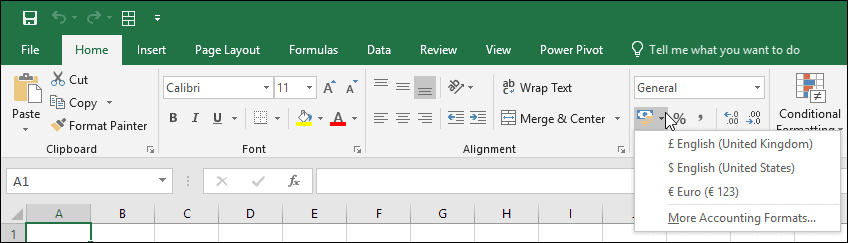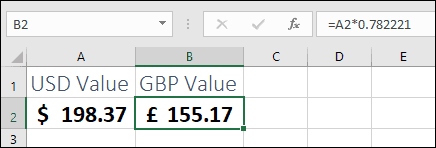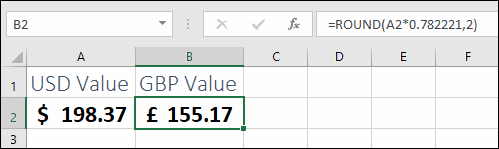# Changing dollar signs to GBP in Excel

## Changing currency symbols in Excel

If all you need to do is change the currency symbol you can do so very easily using the Accounting Number Format drop-down menu on the Excel Ribbon.This drop-down menu only changes the currency symbol; it won’t convert from one currency to another.

When you use this option to apply currency symbols they are applied using a Format. Cell formatting is covered in much greater depth in our Essential Skills Books and E-books.

## Converting currencies in Excel

Actually converting currency values requires the use of a formula. If you’re unfamiliar with Excel formulas you could benefit greatly from our completely free Basic Skills E-book.

To convert a value from USD to GBP at the current exchange rate (at the time I write this) you would use a formula like this:

=A1*0.782221Although the cell formatting makes it look like the value in cell B2 is 155.17, the result of the calculation is actually 155.1691797. This could result in ‘off by one’ errors when you carry out calculations using this data.

When dealing with currency calculations that could result in more than 2 decimal places you should always use the ROUND function to round the result correctly. In this case the formula will be:

=ROUND(A2*0.782221,2)

The ROUND function is covered in greater depth in our Essential Skills Books and E-books.

You can download a copy of the sample workbook to see this formula in action.## Getting exchange rate data from the internet

Exchange rates are constantly changing so you would need to update the formula whenever you need to convert a value. This problem can be overcome by using a Web Query to automatically download exchange rate data from the internet.

Web queries can be created using the Data > Get & Transform Data > Get Data > From Other Sources > From Web option.

You can see an in-depth look at web queries in our Expert Skills Books and E-books.

Scroll to Top